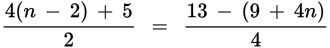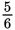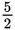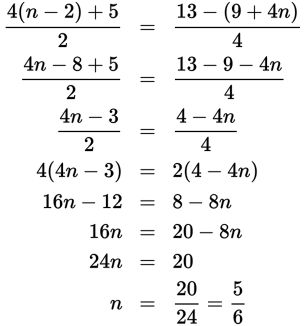# SAT Math Multiple Choice Question 331: Answer and Explanation

### Test Information

Question: 331

1.In the equation above, what is the value of n?

• A.• B.• C. There is no value of n that satisfies the equation.
• D. There are infinitely many values of n that satisfy the equation.

Explanation:

A

Difficulty: Easy

Category: Heart of Algebra / Linear Equations

Strategic Advice: You could start by cross-multiplying to get rid of the denominators, but simplifying the numerators first will make the calculations easier. Don't forget to distribute the negative to both terms in the parentheses on the right-hand side of the equation.